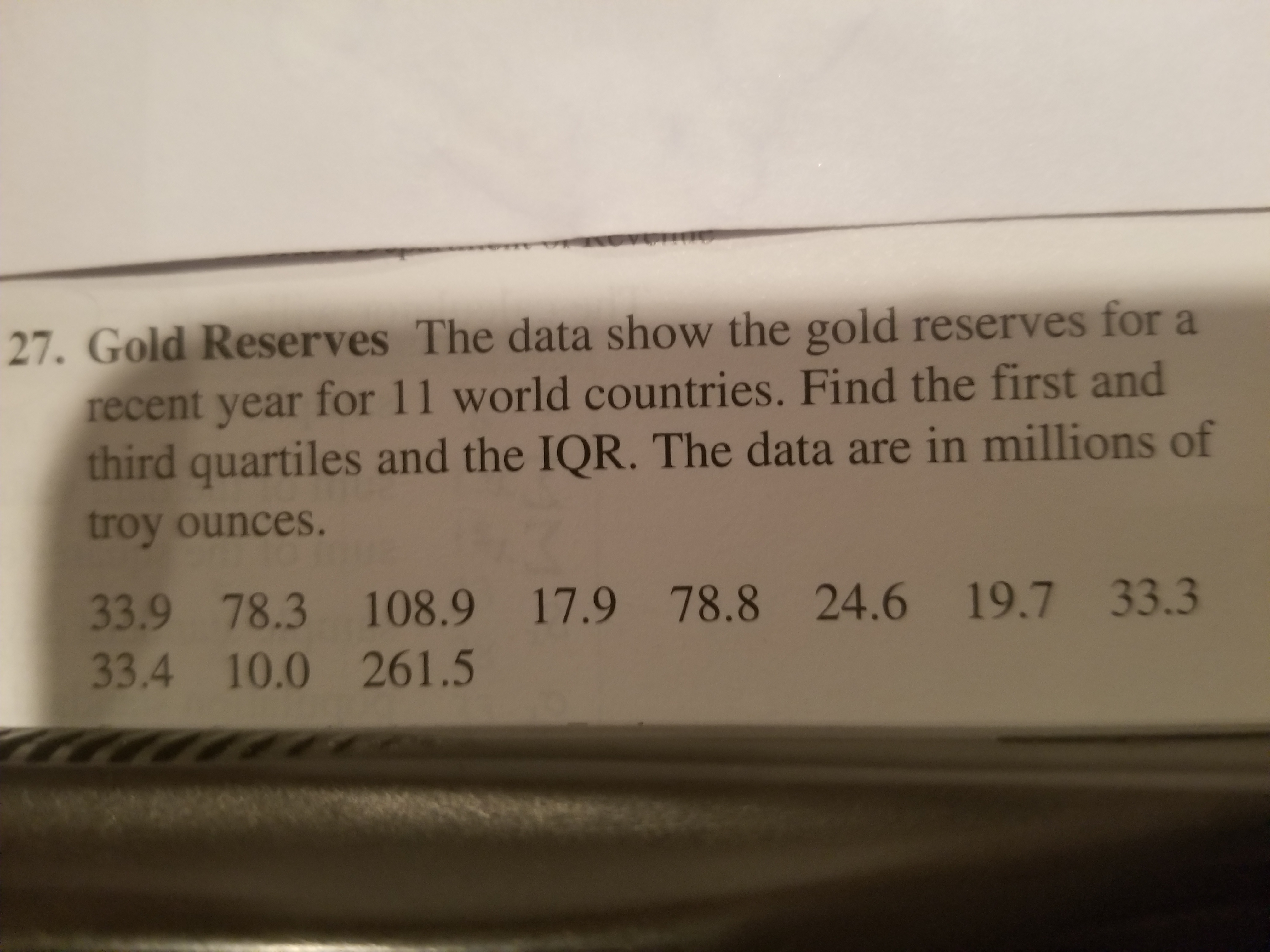# 27. Gold Reserves The data show the gold reserves for arecent year for 11 world countries. Find the first andthird quartiles and the IQR. The data are in millions oftroy ounces.33.9 78.3 108.9 17.9 78.8 24.6 19.7 33.333.4 10.0 261.5

Question
124 viewshelp_outlineImage Transcriptionclose27. Gold Reserves The data show the gold reserves for a recent year for 11 world countries. Find the first and third quartiles and the IQR. The data are in millions of troy ounces. 33.9 78.3 108.9 17.9 78.8 24.6 19.7 33.3 33.4 10.0 261.5 fullscreen
check_circle

Step 1

Obtain the first quartile for the data of gold reserves for a recent year for 11 world countries.

The total number of observations in the data set of gold reserves for a recent year for 11 world countries is n = 11.

The general procedure to obtain first quartile is,

• Arrange the data in ascending order.
• Obtain the value of c = (n/4).
• If the value of c is not a whole number, then round the value of c to the next whole number. The cth term in the ordered dataset is the first quartile of the dataset.
• If the value of c is a whole number, then the first quartile is the average of cth and (c+1)th term in the ordered data set.

The data set of gold reserves for a recent year for 11 world countries is arranged in ascending order as follows:

10, 17.9, 19.7, 24.6, 33.3, 33.4, 33.9, 78.3, 78.8, 108.9 and 261.5

The first quartile for the data of gold reserves for a recent year for 11 world countries is obtained as 19.7 million troy ounces from the calculation given below:

Step 2

The general procedure to obtain third quartile is,

• Arrange the data in ascending order.
• Obtain the value of c = (3n/4).
• If the value of c is not a whole number, then round the value of c to the next whole number. The cth term in the ordered dataset is the third quartile of the dataset.
• If the value of c is a whole number, then the third quartile is the average of cth and (c+1)th term in the ordered data set.

The third quartile for the data of gold reserves for a recent year for 11 world countries is obtained as 78.8 million troy ounces from the calculation given below:

Step 3

Obtain the inert quartile range for the data of gold reserves for a recent year for 11 world countries.

The first quartile for the data of gold reserves for a recent year for 11 world countries is 19.7 million troy ounces.

The third quartile for the data of gold reserves for a recent year for 11 ...

### Want to see the full answer?

See Solution

#### Want to see this answer and more?

Solutions are written by subject experts who are available 24/7. Questions are typically answered within 1 hour.*

See Solution
*Response times may vary by subject and question.
Tagged in

### Statistics